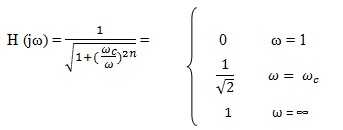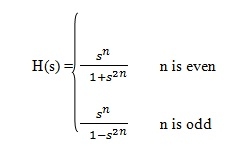# What is a High Pass Filter? Circuit Diagram, Characteristics, and Applications

There was an era where while making a telephone call over distance places, one had to put his mouth very close to the transmitter, speak very slowly and very loudly so that message can be heard clearly by the person at the other end. Today, we can even make video calls over worldwide with high-quality resolutions. The secret of such a tremendous development of technology lies in Electrical filter theory and Transmission line theory. Electrical filters are circuits that pass only selected band of frequencies while attenuating other unwanted frequencies. One of such filters is High pass filter.

## What is a High Pass Filter?

The definition of high pass filter is a filter which passes only those signals whose frequencies are higher than cutoff frequencies thereby attenuating signals of lower frequencies. The value of cutoff frequency depends on the design of the filter.

## High Pass Filter Circuit

The basic High Pass Filter is built by a series connection of capacitor and resistor. While the input signal is applied to the capacitor, the output is drawn across the resistor.

In this circuit arrangement, the capacitor has high reactance at lower frequencies so it acts as an open circuit to the low-frequency input signals until cutoff frequency ‘fc’ is reached. Filter attenuates all the signals below the cutoff frequency level. At frequencies above cut off frequency level reactance of the capacitor becomes low and it acts as a short circuit to these frequencies thereby allowing them to pass directly to the output.

### Passive RC High Pass Filter

The above shown High Pass filter is also known as Passive RC High Pass filter as the circuit is built using only passive elements. There is no need of applying external power for working of the filter. Here capacitor is the reactive element and output is drawn across the resistor.

### High Pass Filter Characteristics

When we talk about cutoff frequency we refer to the point in the frequency response of the filter where the gain is equal to 50% the peak gain of the signal .i.e. 3dB of the peak gain. In High Pass Filter gain increases with an increase in frequencies.

This cutoff frequency fc depends on R and C values of the circuit. Here Time constant τ = RC, the cutoff frequency is inverse proportional to the time constant.

Cutoff frequency = 1/2πRC

Circuit gain is given by AV = Vout/Vin

.i.e. AV = (Vout)/(V in) = R/√(R2 + Xc2) = R/Z

At low frequency f: Xc→∞, Vout = 0

At high-frequency f: Xc →0, Vout = Vin

#### High Pass Filter Frequency Response or High Pass Filter Bode Plot

In high pass filter, all frequencies lying below the cutoff frequency ‘fc’ are attenuated. At this cut off frequency point we get -3dB gain and at this point reactance of the capacitor and resistor values will be same .i.e. R = Xc. Gain is calculated as

Gain (dB) = 20 log (Vout/Vin)

The slope of high pass filter curve is +20 d B/ decade .i.e. after passing cutoff frequency level the output response of the circuit increases from 0 to Vin at a rate of +20 dB per decade which is 6 dB increase per octave.

The region from the initial point to cutoff frequency point is known as stop band as no frequencies are allowed to pass. The region from above the cutoff frequency point. i.e. -3 dB point is known as the passband. At cutoff frequency, point output voltage amplitude will be 70.7% of the input voltage.

Here bandwidth of the filter denotes the value of frequency from which signals are allowed to pass. For example, if the bandwidth of the high pass filter is given as 50 kHz it means that only frequencies from 50 kHz to infinity are allowed to pass.

The phase angle of the output signal is +450 at the cut off frequency. The formula to calculate the phase shift of high pass filter is

∅=arctan ⁡(1/2πfRC)

In practical application, the output response of filter does not extend to infinity. The electrical characteristic of the filter elements applies the limitation to the filter response. By proper selection of filter components, we can adjust the range of frequencies to be attenuated, the range to be passed etc…

### High Pass Filter using Op-Amp

In this high pass filter along with passive filter elements, we add Op-amp to the circuit. Instead of getting an infinite output response, here the output response is limited by open loop characteristics of the Op-amp. Hence this filter acts as a band-pass filter with a cut off frequency which is defined by the bandwidth and gain characteristics of Op-amp.

The open loop voltage gain of Op-amp acts as a limitation to the bandwidth of the amplifier. The gain of the amplifier reduces to 0 dB with the increase in input frequency. The response of the circuit is similar to passive high pass filter but here gain of the Op-amp amplifies the amplitude of the output signal.

The gain of the filter using non inverting Op-amp is given by:

AV = Vout/Vin = (Af (f/fc))/√(1+ (f/fc)^2 )

where Af is passband gain of the filter = 1+( R2)/R1

f is the frequency of the input signal in Hz

fc is the cut off frequency

When low tolerance resistors and capacitors are used these High Pass Active filters provide good accuracy and performance.

### Active High Pass Filter

High Pass Filter using Op-amp is also known as an active high pass filter because along with passive elements capacitor and resistor an active element Op-amp is used in the circuit. Using this active element we can control the cutoff frequency and output response range of the filter.

#### Second Order High Pass Filter

The filter circuits we saw till now are all considered as first order high pass filters. In second order high pass filter, an additional block of an RC network is added to the first order high pass filter at the input path.

### Second Order High Pass Filter

The frequency response of second order high pass filter is similar to the first order high pass filter. But in second order high pass filter stop band will be twice that of first order filter at 40dB/Decade. Higher order filters can be formed by cascading first and second order filters. Although there is no limit to the order, the size of the filter increases along with their order and accuracy degrades. If in higher order filter R1 =R2= R3 etc… and C1=C2=C3= etc…then cutoff frequency will be same regardless of the order of the filter.

The cutoff frequency of second order High Pass Active filter can be given as

fc= 1/(2π√(R3 R4 C1 C2 ))

#### High Pass Filter Transfer Function

As the impedance of the capacitor changes frequently, electronic filters have a frequency-dependent response.

The complex impedance of a capacitor is given as Zc=1/sC

Where,s = σ + jω, ω is the angular frequency in radians per second

The transfer function of a circuit can be found using standard circuit analysis techniques such as Ohm’s law, Kirchhoff’s Laws, Superposition etc. The basic form of a Transfer function is given by the equation

H(s) = (am s^m+a(m-1) s^(m-1)+⋯+a0)/(bn s^n+b(n-1) s^(n-1)+⋯+b0 )

The order of the filter is known by the degree of the denominator. Poles and Zeros of the circuit are extracted by solving roots of the equation. The function may have real or complex roots. The way these roots are plotted on s plane, where σ is denoted by the horizontal axis and ω is denoted by the vertical axis, reveals lots of information about the circuit. For high pass filter, a zero is located at the origin.

H( jω) = Vout/Vin = (-Z2 (jω))/(Z1 (jω))

= – R2/(R1+ 1/jωC)

= -R2/R1 ( 1/(1+ 1/(jωR1 C))

Here H(∞) = R2/R1 , gain when ω →∞

τ = R1 C and ωc= 1/(τ ) .i.e. ωc= 1/(R1C ) is the cut-off frequency

Thus the transfer function of high pass filter is given by H( jω) = – H(∞)( 1/(1+ 1/jωτ))

= – H(∞)( 1/(1- (jωc)/ω))

When input frequency is low then Z1 (jω) is large, therefore the output response is low.

H( jω) = (- H(∞))/√(1+(ωc/ω)^2 ) = 0 when ω=0; H(∞)/√2 when ω=ω_c;

When R1= R2, s = jω and H(0) = 1

So, the transfer function of High Pass Filter H(jω) = jω/(jω+ ω_c )

### Butter worth High Pass Filter

Besides being rejecting the unwanted frequencies, an ideal filter should also have uniform sensitivity for wanted frequencies. Such an ideal filter is impractical. But Stephen Butter worth in his paper “On the theory of filter amplifiers” showed that this type of filter can be achieved by increasing the number of filter elements of right magnitudes.

Butter worth filter is designed in such a way that it gives flat frequency response in the passband of the filter and decreases towards zero in the stop band. A basic prototype of Butter worth filter is the low pass design but by modifications high pass and band pass filters can be designed.

As we have seen above for a first order high pass filter unit gain is H(jω) = jω/(jω+ ω_c)

For n such filters in series H (jω) = (jω/(jω+ ω_c ))^n which upon solving equals to‘n’ controls the order of transition between pass band and stop band. Therefore higher the order, rapid the transition so, at n = ∞ Butter worth filter becomes an ideal High Pass Filter.

During the implementation of this filter for simplicity we consider ωc = 1 and solve the transfer function

for s = jω .i.e. H(s) = s/(s+ωc ) = s/(s+1) for order 1:

H(s) = s^2/(s^2+∆ωs+(ωc^2 ) for order 2

Therefore the transfer function of the cascade in High Pass Filter is